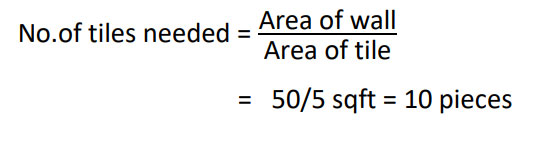# How do you calculate the number of tiles you need ?

Tile, manufactured from clay, porcelain, or stone, is available in an ever-changing variety of sizes, shapes, colors, textures, patterns, finishes, and thicknesses. Tile provides a hard, durable, and virtually maintenance-free surface for both interior and exterior use.

If you're planning on installing your own tiles on floor or simply calculating the material you need to budget for , you can determine how much tiles you need , whether it's a wall project or a floor project.

With a measuring tape and some simple math , you can estimate how much tiles you need and how much will the project cost you.

### WHAT WILL YOU NEED:

• PAPER
• PEN OR PENCIL
• MEASURING TAPE
• CALCULATOR (optional)

## 5 STEPS CALCULATING ON HOW MUCH TILES YOU WILL NEED.

###### STEP 1- Measure the length of one side of the room.

If it is a wall, measure the length of the wall up to down. Let's name the wall A and it's length is 10 feet.

###### STEP 2 ? Measure the length of the other side of the room

If it's a wall, measure the length of the wall from left to right. Let's name the wall as B and it's length is 5 feet.

###### STEP 3- Multiple the two measurements.

A = 10ft. B = 5ft.

Therefore, AREA OF WALL= A ? B

= 10?5 =50sqft

###### STEP 4 ? Convert the square footage to the amount of tiles you need

Tiles comes in boxes and you have to buy the whole box. Divide the total square footage of the room by the total square footage of the tile in the box.

Here we are giving you the area of tile as 5 square feet.

To find the number of tiles to be filled:###### STEP 5 ? Calculate the wastage you will need

You should never buy the exact amount of tiles you need. Sometimes you need a bit extra for cuts, waste, breaks or mistakes.

Let's assume standard wastage is 5%.

So, {10 + (5% of 10)} = 10.5 pcs.

To get more clear idea, go through the following video tutorial